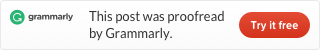Categories

# Leetcode problem 240 Search a 2D Matrix II (Java)

Write an efficient algorithm that searches for a value in an m x n matrix. This matrix has the following properties:

• Integers in each row are sorted in ascending from left to right.
• Integers in each column are sorted in ascending from top to bottom.

Example:

Consider the following matrix:

```[
[1,   4,  7, 11, 15],
[2,   5,  8, 12, 19],
[3,   6,  9, 16, 22],
[10, 13, 14, 17, 24],
[18, 21, 23, 26, 30]
]```

Given target = `5`, return `true`.
Given target = `20`, return `false`.

This problem we tried 4 solutions, vertical and “horizontal binary search” approach,vertical and horizontal both binary search” approach, 4 area binary search approach, sort then binary search approach.

## Vertical and “horizontal binary search” approach

This is a direct approach, is a divide and conquer way. First, we cut the matrix vertically to the top and bottom two parts. Then cut, again and again, until there is only one line of numbers, we use a binary search code to search this line. This way looks like a mergesort+binary search.

Running time is 52ms, not fast enough. This because we only use one property of the matrix, ascending from left to right, we didn’t use the property of ascending from top to bottom.

## “Vertical and horizontal both binary search”

We need to use binary search in both ways to be faster. When we cut the matrix, we can find out two key points, one is the right-bottom corner p1 of the top sub-matrix, if target bigger than it, we don’t need to search the top sub-matrix. Another one is the left-top corner of the bottom sub-matrix p2 if the target is smaller than it, we don’t search it either. Like this:

In the code p1 is matrix[mid][matrix[mid].length-1], p2 is matrix[mid+1]. So the code like this:

Running time is 27ms still not fast enough.

## 4 areas search

I know we can split the matrix into 4 areas to search, but I try to avoid this way. Because code will be really messy. Now we know last way is not fast enough, I have to code in this way. It is not complex to think, cut the matrix into 4 areas, then cut and cut, until there is only one element. We can use the key points (every left-top and right bottom corner of sub-matrixes) to seep up searches.

This thought is not hard, but the code is very complex. You may already find out this is very similar to Strassen’s algorithm for matrix multiplication from Introduction to Algorithms. This is the code:

Running time is 7ms is fast enough.

## Sort then binary search approach

All these solutions are too complex, can we solve it easily? I tried once expend the matrix to an array, then use a binary search. But I find out the matrix ascending from left to right and ascending from top to bottom, don’t mean it can expend to an ordered array. So directly use a binary search will not get the correct result.

So we only can expend the matrix to an array, and sort it, then use a binary search. The code like this:

Code is very simple but very slow, running time is 610ms, is not very useful. The 4 area solution is best.

Want to know details of the Divide and conquer, check my post: Leetcode problems of Divide and conquer.This site uses Akismet to reduce spam. Learn how your comment data is processed.